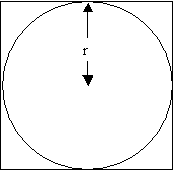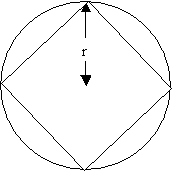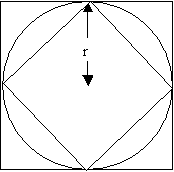#### You may also like### Shades of Fermat's Last Theorem

The familiar Pythagorean 3-4-5 triple gives one solution to (x-1)^n + x^n = (x+1)^n so what about other solutions for x an integer and n= 2, 3, 4 or 5?### Two Cubes

Two cubes, each with integral side lengths, have a combined volume equal to the total of the lengths of their edges. How big are the cubes? [If you find a result by 'trial and error' you'll need to prove you have found all possible solutions.]### Exhaustion

Find the positive integer solutions of the equation (1+1/a)(1+1/b)(1+1/c) = 2

# Approximating Pi

##### Age 14 to 18Challenge Level

By inscribing a circle in a square and then a square in a circle find an approximation to pi.
By using a hexagon, can you improve on the approximation? How much better an approximation is it?

Archimedes used this idea first with a hexagon, then a dodecagon (12 sides) and so on up to a 96 sided polygon to calculate pi and was able to establish that $$3\frac{10}{71} < \pi < 3 \frac{1}{7}$$

What are the strengths and limitations of this method?